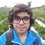# Pre-RMO 2014/18

Let $f$ be a one-to-one function from the set of natural numbers to itself such that $f(mn) = f(m)f(n)$ for all natural numbers $m$ and $n$. What is the least possible value of $f(999)$?

This note is part of the set Pre-RMO 2014Note by Pranshu Gaba
6 years ago

This discussion board is a place to discuss our Daily Challenges and the math and science related to those challenges. Explanations are more than just a solution — they should explain the steps and thinking strategies that you used to obtain the solution. Comments should further the discussion of math and science.

When posting on Brilliant:

• Use the emojis to react to an explanation, whether you're congratulating a job well done , or just really confused .
• Ask specific questions about the challenge or the steps in somebody's explanation. Well-posed questions can add a lot to the discussion, but posting "I don't understand!" doesn't help anyone.
• Try to contribute something new to the discussion, whether it is an extension, generalization or other idea related to the challenge.

MarkdownAppears as
*italics* or _italics_ italics
**bold** or __bold__ bold
- bulleted- list
• bulleted
• list
1. numbered2. list
1. numbered
2. list
Note: you must add a full line of space before and after lists for them to show up correctly
paragraph 1paragraph 2

paragraph 1

paragraph 2

[example link](https://brilliant.org)example link
> This is a quote
This is a quote
    # I indented these lines
# 4 spaces, and now they show
# up as a code block.

print "hello world"
# I indented these lines
# 4 spaces, and now they show
# up as a code block.

print "hello world"
MathAppears as
Remember to wrap math in $$ ... $$ or $ ... $ to ensure proper formatting.
2 \times 3 $2 \times 3$
2^{34} $2^{34}$
a_{i-1} $a_{i-1}$
\frac{2}{3} $\frac{2}{3}$
\sqrt{2} $\sqrt{2}$
\sum_{i=1}^3 $\sum_{i=1}^3$
\sin \theta $\sin \theta$
\boxed{123} $\boxed{123}$

Sort by:

We can write $f(999) = (f(3))^3 \cdot f(37)$

To minimize this expression we can define the function $f$ as follows:

If $x$ is composite, it must be prime factorized, as $f(999)$ is factorized above. Then:

$f(x) = \begin{cases} 1 & x = 1 \\ 37 & x = 2 \\ 2 & x = 3 \\ 3 & x = 37 \\ p & x = p, p \text{ is a prime} \neq 2, 3, 37\end{cases}$

This gives $f(999) = 8 \times 3 = \boxed{24}$.

- 6 years ago

Good. But you need to justify that this function is one-one. This is not required in the exam, but still as a matter of learning, one should leave no loop hole.

- 5 years, 12 months ago

It is clearly justified, isn't it. For 1,2,3,37, it is visible that it is one -one. For all numbers other than 2, 3, 37 it is one-one because if the number is prime then it maps the prime number to itself hence one-one, and if it is composite then it can be written as a product of its primes which will yield product of one-one functions which is one-one itself.(as the function gives unique primes).

- 2 years, 4 months ago

thanks Geeta

- 2 years, 2 months ago

$f(x) = x^{n}$ (n is odd number)

Least possible value of

$f(999) = 0, \text{when n }\to -\infty$

- 6 years ago

I don't think $f(x) = x^n$ is a one-to-one function, as not all natural numbers are included in the range of $f(x)$. Also, if $n$ is negative, $f(x)$ will be a fraction, but we want it to be a natural number.

- 6 years ago

$f(x)=x^n$ is indeed a one-one function if $n$ is odd, but it is not mapped on set of Natural numbers if $n$ is negative, so this function does not satisfy the given conditions.

- 5 years, 12 months ago